## The Laplace Transform Operator

A particular kind of integral transformation is known as the Laplace transformation, denoted by L. The definition of this operator is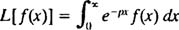The result—called the Laplace transform of f—will be a function of p, so in general,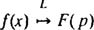Example 1: Find the Laplace transform of the function f( x) = x.

By definition,Integrating by parts yieldsTherefore, the function F( p) = 1/ p 2 is the Laplace transform of the function f( x) = x. [Technical note: The convergence of the improper integral here depends on p being positive, since only then will ( x/p) e px and e px approach a finite limit (namely 0) as x → ∞. Therefore, the Laplace transform of f( x) = x is defined only for p > 0.]

In general, it can be shown that for any nonnegative integer n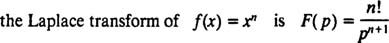Like the operators D and I—indeed, like all operators—the Laplace transform operator L acts on a function to produce another function. Furthermore, since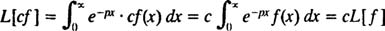andthe Laplace transform operator L is also linear.

[Technical note: Just as not all functions have derivatives or integrals, not all functions have Laplace transforms. For a function f to have a Laplace transform, it is sufficient that f( x) be continuous (or at least piecewise continuous) for x ≥ 0 and of exponential order (which means that for some constants c and λ, the inequalityholds for all x). Any bounded function (that is, any function f that always satisfies | f( x)| ≤ M for some M ≥ 0) is automatically of exponential order (just take c = M and λ = 0 in the defining inequality). Therefore, sin kx and cos kx each have a Laplace transform, since they are continuous and bounded functions. Furthermore, any function of the form e kx , as well as any polynomial, is continuous and, although unbounded, is of exponential order and therefore has a Laplace transform. In short, most of the functions you are likely to encounter in practice will have Laplace transforms.]

Example 2: Find the Laplace transform of the function f( x) = x 3 – 4 x + 2.

Recall form the first statement following Example 1 that the Laplace transform of f( x) = x n is F( p) = n!/ p n + 1 . Therefore, since the Laplace transform operator L is linear,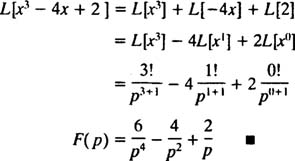Example 3: Determine the Laplace transform of f( x) = e kx .

Apply the definition and perform the integration: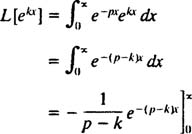In order for this improper integral to converge, the coefficient ( pk) in the exponential must be positive (recall the technical note in Example 1). Thus, for p > k, the calculation yieldsExample 4: Find the Laplace transform of f( x) = sin kx.

By definition,This integral is evaluated by performing integration by parts twice, as follows: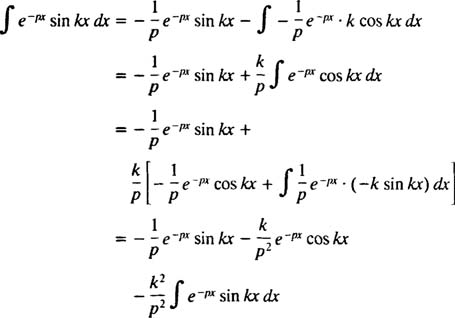so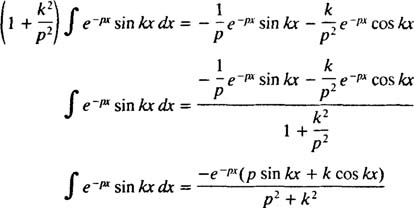Therefore,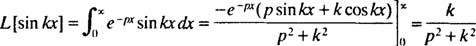for p > 0. By a similar calculation, it can be shown that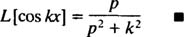Example 5: Determine the Laplace transform of the function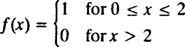pictured in Figure 1:Figure 1

This is an example of a step function. It is not continuous, but it is piecewise continuous, and since it is bounded, it is certainly of exponential order. Therefore, it has a Laplace transform.Table 1 assembles the Laplace transforms of a few of the most frequently encountered functions, as well as some of the important properties of the Laplace transform operator L.Example 6: Use Table 1 to find the Laplace transform of f( x) = sin 2 x.

Invoking the trigonometric identity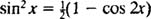linearity of L impliesExample 7: Use Table 1 to find the Laplace transform of g( x) x 3 e 5x.

The presence of the factor e 5x suggests using the shifting formula with k = 5. Sincethe shifting formula says that the Laplace transform of f( x) e 5x = x 3 e 5x is equal to F( P – 5). In other words, the Laplace transform of x 3 e 5x is equal to the Laplace transform of x 3 with the argument p shifted to p – 5: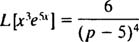Example 8: Use Table 1 to find Laplace transform of f( x) = e −2x sin x – 3.

First, since L [sin x] = 1/( p 2 + 1), the shifting formula (with k = −2) saysNow, because L = 3 · L = 3/ p, linearity implies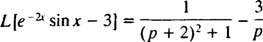Example 9: Use Table 1 to find a continuous function whose Laplace transform is F( p) = 12/ p 5.

This example introduces the idea of the inverse Laplace transform operator,, L −1. The operator L −1 will “un‐do” the action of L. Symbolically,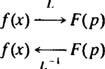If you think of the operator L as changing f( x) into F( p), then the operator L −1 just changes F( P) back into f( x). Like L, the inverse operator L −1 is linear.

More formally, the result of applying L −1 a function F( p) is to recover the continuous function f( x) whose Laplace transform is the given F( p). [This situation should remind remind you of the operators D and I (which are, basically, inverses of one another). Each will un‐do the action of the other in the sense that if, say, I changes f( x) into F( x), then D will change F( x) back into f( x). In other words, D = I −1, so if you apply I and then D, you're back where you started.]

Using Table 1 (reading it from to left),Example 10: Find the continuous function whose Laplace transform is F( p) = 1/( p 2 – 1).

By partial fraction decomposition,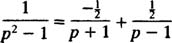Therefore, by linearity of L −1,Example 11: DetermineFirst, note that p has been shifted to p + 2 = p – (‐2). Therefore, since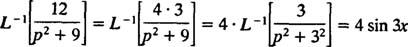the shifting formula (with k = −2) impliesExample 12: Evaluate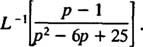Although p 2 – 6 p + 25 cannot be factored over the integers, it can be expressed as the sum of two squares: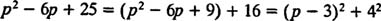Therefore,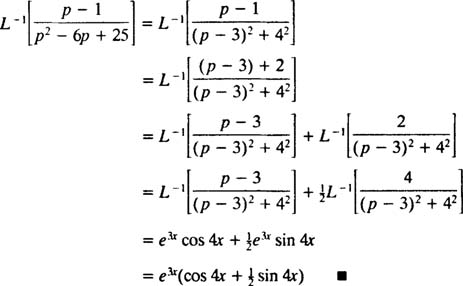Back to Top
A18ACD436D5A3997E3DA2573E3FD792A